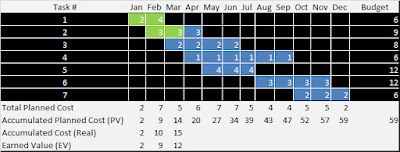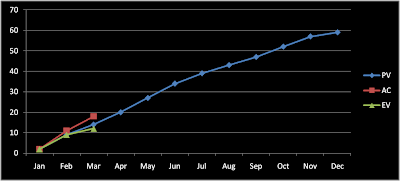### Earned Value

The Earned Value (EV) is a function of the time and it represents the progress of a project. It's measured in units of economic value (\$). Mathematically, the domain of the Earned Value (EV) is time and the range is economic value (\$). The Earned Value (EV) is clearly associated with the Planned Value (PV) because the value that EV has, when a milestone is passed (or a goal is achieved), is the same that PV has in the same circunstances. In other words, when a unit of work has be done, EV adds the same value that was planned for that unit of work in curve PV, no matter how much was the cost of finishing that unit of work.

In the folowing table could see an example. Suppose that we finished the first task, we are progressing in the second and the third hasn't been started. The real cost exceeds the budget. The earned value is composed of the completed work, valuated with the planned cost.Click to maximize
It's a convention that the Earned Value (EV) starts at the time zero and goes until the real duration of the project. The minimun value is zero and the maximun value of the Earned Value (EV) is the total budget of the project. It's possible to see the last example drawed in the folowing curves.Click to maximize